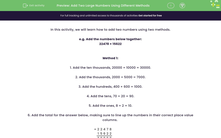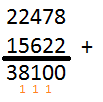### Comprehensive & curriculum aligned

In this worksheet, students add two numbers with four digits or more using two different methods.Key stage:  KS 2

Curriculum topic:   Number: Addition and Subtraction

Curriculum subtopic:   Add/Subtract Four-Digit (or more) Numbers

Popular topics:   Subtraction worksheets, Addition worksheets, Place Value worksheets

Difficulty level:#### Worksheet Overview

In this activity, we will learn how to add two numbers using two methods.

e.g. Add the numbers below together:

22478 + 15622

Method 1:

1. Add the ten thousands, 20000 + 10000 = 30000.

2. Add the thousands, 2000 + 5000 = 7000.

3. Add the hundreds, 400 + 600 = 1000.

4. Add the tens, 70 + 20 = 90.

5. Add the ones, 8 + 2 = 10.

6. Add the total for the answer below, making sure to line up the numbers in their correct place value columns.

 + 2 2 4 7 8 1 5 6 2 2 3 0 0 0 0 7 0 0 0 1 0 0 0 9 0 1 0 3 8 1 0 0

Method 2:

This is column addition with exchanging if a column adds to 10 or more.

1. Add the ones, 8 + 2 = 10. Write the 0 in the ones column, and exchange the 1 under the tens column.

2. Add the tens column, including the exchanged 1. Add 7 + 2 + 1 = 10. Write the 0 in the tens column and exchange the 1 under the hundreds column.

3. Add the hundreds column, including the exchanged 1. Add 4 + 6 + 1 = 11. Write the 1 in the hundreds column and exchange the 1 under the thousands column.

4. Add the thousands column, including the exchanged 1. Add 5 + 2 + 1 = 8.

5. Add the ten thousands column, 2 + 1 = 3.So in summary:

22478 + 15622 = 38100

For the questions in this activity, you can choose which method you prefer, or have a go at using both!

### What is EdPlace?

We're your National Curriculum aligned online education content provider helping each child succeed in English, maths and science from year 1 to GCSE. With an EdPlace account you’ll be able to track and measure progress, helping each child achieve their best. We build confidence and attainment by personalising each child’s learning at a level that suits them.

Get started